## Count unique numeric values or dates in a column

Supposing you have a list of numbers which contain some duplicates, now, you want to count the number of the unique values or the values appear only once in the list as below screenshot shown. In this article, we will talk about some useful formulas for solving this task in Excel quickly and easily.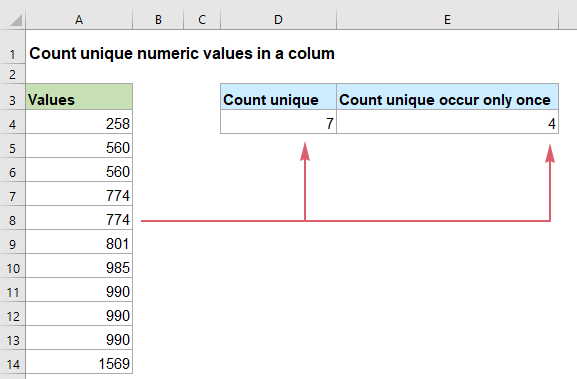#### Count unique numeric values or dates in a column in Excel 2019, 2016 and earlier

If you are using Excel 2019, 2016 and earlier versions, you can apply the normal SUM, FREQUENCY, IF, COUNTIF, ISNUMBER functions to get the number of unique values or the values that occur only once.

##### Count unique numeric values or dates in a column

To count unique numbers from a list, the SUM and FREQUENCY functions may do you a favor, the generic syntax is:

=SUM(--(FREQUENCY(range,range)>0))
• range: The data column where you want to count unique values from.

Please enter or copy the following formula into a blank cell to output the result, and then press Enter key to get the result:

=SUM(--(FREQUENCY(A2:A12,A2:A12)>0))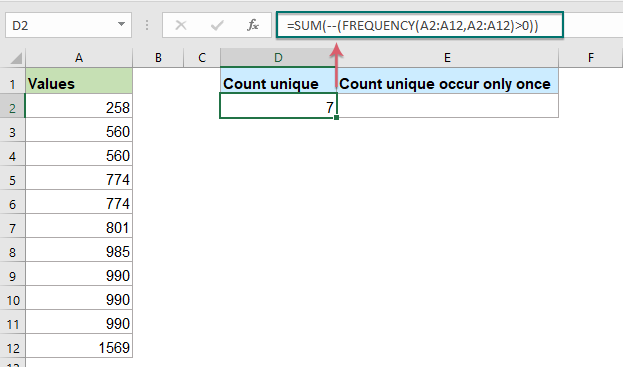##### Explanation of the formula:

=SUM(--(FREQUENCY(A2:A12,A2:A12)>0))

• FREQUENCY(A2:A12,A2:A12): The FREQUENCY function returns the count of each numeric value in the list A2:A12, and it will get an array like this: {1;2;0;2;0;1;1;3;0;0;1;0}. The numbers in the array indicate that how many times the values appear in the column. And the number 0s in the array means that the numbers have been already appeared in the data range.
• --(FREQUENCY(A2:A12,A2:A12)>0=--({1;2;0;2;0;1;1;3;0;0;1;0})>0: Each value in the array will be compared to zero: if greater than 0, a TRUE is displayed, otherwise, a FALSE is displayed. And then, the double negative sign converts the TRUEs and FALSEs to 1s and 0s, so, it will return the result as this: {1;1;0;1;0;1;1;1;0;0;1;0}.
• SUM(--(FREQUENCY(A2:A12,A2:A12)>0))=SUM({1;1;0;1;0;1;1;1;0;0;1;0}): At last, the SUM function sums all the values in the array, and get the result: 7.

##### Count unique numeric values or dates that appear only once in a column

Count the unique numeric values that appear only once from a list, you should combine the SUM, IF, ISNUMBER and COUNTIF functions to create the formula, the generic syntax is:

{=SUM(IF(ISNUMBER(range)*COUNTIF(range,range)=1,1,0))}
Array formula, should press Ctrl + Shift + Enter keys together.
• range: The data column where you want to count unique values from.

Now, please enter or copy the below formula into a blank cell, and then press Ctrl + Shift + Enter keys simultaneously to get the correct result:

=SUM(IF(ISNUMBER(A2:A12)*COUNTIF(A2:A12,A2:A12)=1,1,0))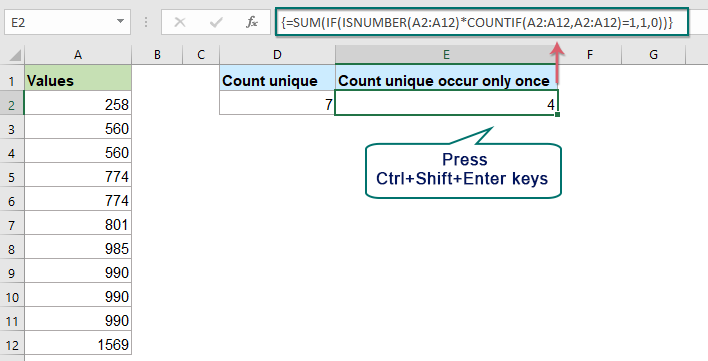##### Explanation of the formula:

=SUM(IF(ISNUMBER(A2:A12)*COUNTIF(A2:A12,A2:A12)=1,1,0))

• ISNUMBER(A2:A12): This ISNUMBER function is used to check the values in the list A2:A12 if they are numbers or not. It will return TRUE if a cell contains a numeric value, and FALSE if not. So, you will get an array like this: {TRUE;TRUE;TRUE;TRUE;TRUE;TRUE;TRUE;TRUE;TRUE;TRUE;TRUE}.
• COUNTIF(A2:A12,A2:A12): The COUNTIF function counts how many times each value appears in the specific range, it will return the result as this: {1;2;2;2;2;1;1;3;3;3;1}.
• ISNUMBER(A2:A12)*COUNTIF(A2:A12,A2:A12)= {TRUE;TRUE;TRUE;TRUE;TRUE;TRUE;TRUE;TRUE;TRUE;TRUE;TRUE}* {1;2;2;2;2;1;1;3;3;3;1}: These two expressions are multiplied to get this result: {1;2;2;2;2;1;1;3;3;3;1}.
• IF(ISNUMBER(A2:A12)*COUNTIF(A2:A12,A2:A12)=1,1,0)=IF({1;2;2;2;2;1;1;3;3;3;1}=1,1,0): Here the IF function checks each value in the array if they are equal to 1 or not: If yes, a number 1 will be returned; if not, a number 0 is displayed. The result is like this: {1;0;0;0;0;1;1;0;0;0;1}.
• SUM(IF(ISNUMBER(A2:A12)*COUNTIF(A2:A12,A2:A12)=1,1,0))=SUM({1;0;0;0;0;1;1;0;0;0;1}): Finally, the SUM function adds up the values in the array, and return the total number of the unique values: 4.

#### Count unique numeric values or dates in a column in Excel 365

In Excel 365, there is a new easy function called UNIQUE. To have this function enclosed in the COUNT function can help you deal with this task quickly as you need.

##### Count unique numeric values or dates in a column

The generic syntax to count the unique numeric values or dates in Excel 365 is:

=COUNT(UNIQUE(range))
• range: The data column where you want to count unique values from.

Please enter or copy the below formula into a blank cell and press Enter key to get the result, see screenshot:

=COUNT(UNIQUE(A2:A12))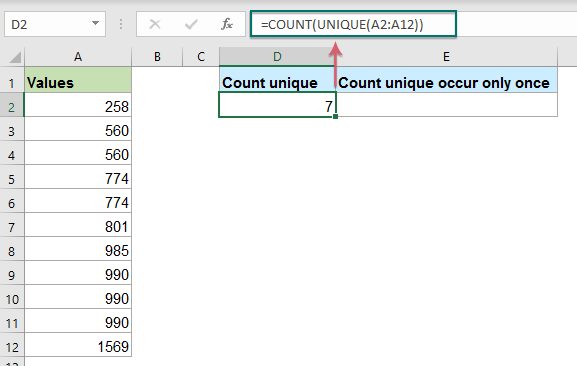##### Explanation of the formula:

=COUNT(UNIQUE(A2:A12))

• UNIQUE(A2:A12): This UNIQUE function extracts all the unique values from the list, and you will get an array list as this: {258;560;774;801;985;990;1569}.
• COUNT(UNIQUE(A2:A12))=COUNT({258;560;774;801;985;990;1569}): This COUNT function counts the number of numeric values and return the result: 7.

##### Count unique numeric values or dates that appear only once in a column

This UNIQUE function also can extract the unique values that occur exactly once from a list, the generic syntax is:

=COUNT(UNIQUE(range,0,1))
• range: The data column where you want to count unique values from.

Enter or copy the following formula into a cell where you want to return the result, and then press Enter key, see screenshot:

=COUNT(UNIQUE(A2:A12,0,1))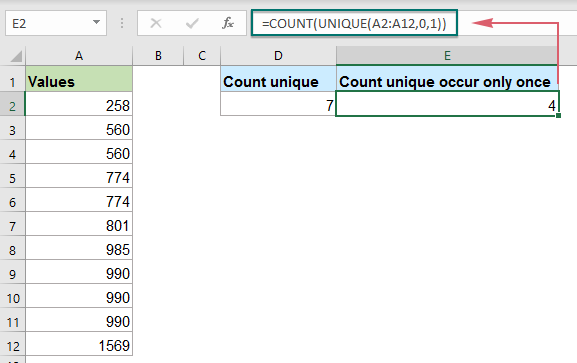##### Explanation of the formula:

=COUNT(UNIQUE(A2:A12,0,1))

• UNIQUE(A2:A12,0,1): This UNIQUE function extracts the unique values only appear once from the list, and you will get an array list as this: {258;801;985;1569}.
• COUNT(UNIQUE(A2:A12,0,1))=COUNT({258;801;985;1569}): This COUNT function counts the number of numeric values and returns the result: 4.

Tips: All above formulas are available for counting the unique dates in a column.

#### Relative function used:

• SUM:
• The Excel SUM function returns the sum of values supplied.
• FREQUENCY:
• The FREQUENCY function calculates how often values occur within a range of values, and then returns a vertical array of numbers.
• ISNUMBER:
• The Excel ISNUMBER function returns TRUE when a cell contains a number, and FALSE if not.
• COUNTIF:
• The COUNTIF function is a statistical function in Excel which is used to count the number of cells that meet a criterion.
• COUNT:
• The COUNT function is used to count the number of cells that contain numbers, or count the numbers in a list of arguments.
• UNIQUE:
• The UNIQUE function returns a list of unique values in a list or range.

#### More articles:

• Count Unique Numeric Values Based On Criteria
• In Excel worksheet, you may suffer from a problem that count the number of unique numeric values based on a specific condition. For example, how can I count the unique Qty values of the product “T-shirt” from the report as below screenshot shown? In this article, I will show some formulas to achieve this task in Excel.
• Count Number Of Cells Equals To One Of Many Values
• Supposing, I have a list of products in column A, now, I want to get the total number of specific products Apple, Grape and Lemon which listed in range C4:C6 from column A as below screenshot shown. Normally, in Excel, the simple COUNTIF and COUNTIFS functions will not work in this scenario. This article, I will talk about how to solve this job quickly and easily with the combination of SUMPRODUCT and COUNTIF functions.
• Count Number Of Cells That Contain Specific Number Of Characters
• If you want to count the number of cells that contain a certain number of characters (such as 5 characters) in a range of cells as below screenshot shown. In Excel, the COUNTIF function can do you a favor. In this article, I will talk about how to get number of cells that contain exactly x characters or cells that contain more than a certain number of characters.

### The Best Office Productivity Tools

#### Kutools for Excel - Helps You To Stand Out From Crowd

 Popular Features: Find/Highlight/Identify Duplicates  |  Delete Blank Rows  |  Combine Columns or Cells without Losing Data  |  Round without Formula ... Super VLookup: Multiple Criteria  |  Multiple Value  |  Across Multi-Sheets  |  Fuzzy Lookup... Adv. Drop-down List: Easy Drop Down List  |  Dependent Drop Down List  |  Multi-select Drop Down List... Column Manager: Add a Specific Number of Columns  |  Move Columns  |  Toggle Visibility Status of Hidden Columns  |  Compare Columns to Select Same & Different Cells ... Featured Features: Grid Focus  |  Design View  |  Big Formula Bar  |  Workbook & Sheet Manager | Resource Library (Auto Text)  |  Date Picker  |  Combine Worksheets  |  Encrypt/Decrypt Cells  |  Send Emails by List  |  Super Filter  |  Special Filter (filter bold/italic/strikethrough...) ... Top 15 Toolsets:  12 Text Tools (Add Text, Remove Characters ...)  |  50+ Chart Types (Gantt Chart ...)  |  40+ Practical Formulas (Calculate age based on birthday ...)  |  19 Insertion Tools (Insert QR Code, Insert Picture from Path ...)  |  12 Conversion Tools (Numbers to Words, Currency Conversion ...)  |  7 Merge & Split Tools (Advanced Combine Rows, Split Cells ...)  |  ... and more

Kutools for Excel Boasts Over 300 Features, Ensuring That What You Need is Just A Click Away...

Supports Office/Excel 2007-2021 and 365   |   Available in 44 Languages   |   Easy to Uninstall Completely#### Office Tab - Enable Tabbed Reading and Editing in Microsoft Office (include Excel)

• One second to switch between dozens of open documents!
• Reduce hundreds of mouse clicks for you every day, say goodbye to mouse hand.
• Increases your productivity by 50% when viewing and editing multiple documents.
• Brings Efficient Tabs to Office (include Excel), Just Like Chrome, Edge and Firefox.